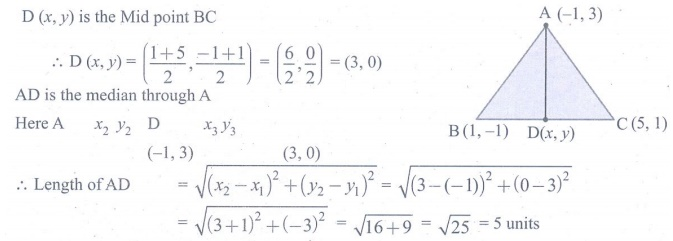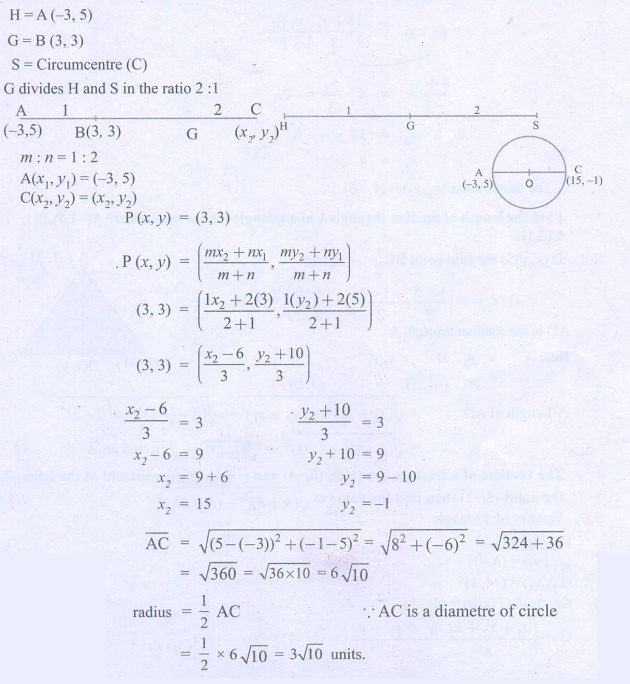Home | | Maths 9th std | Exercise 5.5: The Coordinates of the Centroid

# Exercise 5.5: The Coordinates of the Centroid

Maths : Coordinate Geometry: Book Back, Exercise, Example Numerical Question with Answers, Solution: Exercise 5.5: The Coordinates of the Centroid

Coordinate Geometry: The Coordinates of the Centroid

Exercise 5.5

1. Find the centroid of the triangle whose vertices are

(i) (2,−4), (−3,−7) and (7,2) (ii) (−5,−5), (1,−4) and (−4,−2)2. If the centroid of a triangle is at (4,−2) and two of its vertices are (3,−2) and (5,2) then find the third vertex of the triangle.3. Find the length of median through A of a triangle whose vertices are A(−1,3), B(1,−1) and C(5,1).4. The vertices of a triangle are (1,2), (h,−3) and (−4,k). If the centroid of the triangle is at the point (5,−1) then find the value of √ { (h + k)2 + (h + 3k)2 } .5. Orthocentre and centroid of a triangle are A(−3,5) and B(3,3) respectively. If C is the circumcentre and AC is the diameter of this circle, then find the radius of the circle.6. ABC is a triangle whose vertices are A(3, 4), B(2, 1) and C(5, 3) . If G is the centroid and BDCG is a parallelogram then find the coordinates of the vertex D.7. If  (3/2, 5) , (7, −9/2) and (13/2, −13/2) are mid-points of the sides of a triangle, then find the centroid of the triangle.Tags : Numerical Problems with Answers, Solution | Coordinate Geometry | Maths , 9th Maths : UNIT 5 : Coordinate Geometry
Study Material, Lecturing Notes, Assignment, Reference, Wiki description explanation, brief detail
9th Maths : UNIT 5 : Coordinate Geometry : Exercise 5.5: The Coordinates of the Centroid | Numerical Problems with Answers, Solution | Coordinate Geometry | Maths